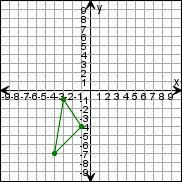Create a new printableGeometry
Math Worksheets

Sample - Click above to make a new math worksheet (PDF).
 Name _____________________________Date ___________________
Transformations
Draw the triangle after the transformations.

 1 * This is a pre-made sheet.Use the link at the top of the page for a printable page.
 2 Translate the triangle left 4 units, then 3 units down. Then rotate the new triangle 90clockwise around (0,0).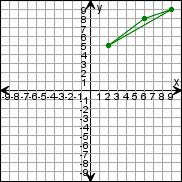3 Translate the triangle up 1 unit, then 2 units right. Then rotate the new triangle 90clockwise around (0,0).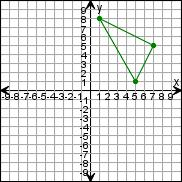4 Translate the triangle up 2 units, then 3 units right. Then rotate the new triangle 180clockwise around (0,0).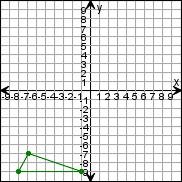5 Translate the triangle right 1 unit, then 4 units down. Then rotate the new triangle 90clockwise around (0,0).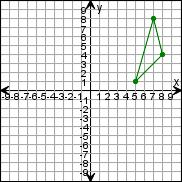6 Translate the triangle right 5 units, then 2 units down. Then rotate the new triangle 180clockwise around (0,0).